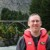# Question re Z-score to Estimate Quantity of Defective Units

Six Sigma – iSixSigma Forums General Forums Tools & Templates Question re Z-score to Estimate Quantity of Defective Units

Viewing 4 posts - 1 through 4 (of 4 total)
• Author
Posts
• #240402Ddelisle
Participant

I sent this discussion earlier today but cannot find it on the forum… not sure what happened.

Using this partial Z Table, how many units from a month’s production run are expected to not satisfy customer requirements for the following process?

Upper specification limit: 8.4 Lower specification limit: 4.7 Mean of the process: 6.2 Standard Deviation: 2.2 Monthly production: 360 units

Response:  57 (from test service)

I approached this question by looking the z-score for upper and lower limits and adding the two to get the total estimate.

My calc:

UCL:  (8.4-6.2)/2.2 = 1.00 (z-score). I change the sign (-1.00)  to get the area to the left and come up with .1587  .1587*360=57

LCL

UCL:  (4.7-6.2)/2.2 = -.68 (z-score).  Z-score = .2483*360=89.4

Add the 89+57 = 146 for a total estimated defects.  I know that this answer does not make sense since we are within one std on upper side and more than one on lower side.  So defects would be less than 115 (1-.68)*360.

So where am I going wrong?

Thanks

0
#240404Chris Seider
Participant

I don’t understand your last paragraph. You have more defective on the lower side than on the upper side so you’d expect to have more than the normal distribution of 31.7% defective since you produce a higher defect rate on the lower side.  Draw the normal curve, place the specs and darken the defective areas.  Then do the same for dark areas for +/- 1 s.d. out of spec–which has more.

0
#240433Ddelisle
Participant

Hi Chris-

In regards to the last paragraph…looking at this again,  I was wrong the LCL is less than 1 sigma which would yield a higher defect rate.  So my calculation of 146 still hold true.  Assuming the Z-score I picked up the correct rates on the Z-score table, then I my answer should be correct… ?

Tks

Derek

0
#255102VazBelt
Participant

I was struggling with the same question when I came across this topic. I though it’s best to share my findings.

1. Z (ucl) = 1 > Area to the left of UCL = 0.8413 (from table)

2. Since we want the area to the right of UCL (upper rejection zone) : 1 – 0.8413 = 0.1586 — A

3. Z (lcl) = -0.6818 > Area to the left of LCL = 0.2483 (from table) — B

4. So the rejection areas = 0.1586 + 0.2483 = 0.407 — A + B

5. This means that 40.7% of the production run are expected to not satisfy customer = 40.7% of 360 units = 146